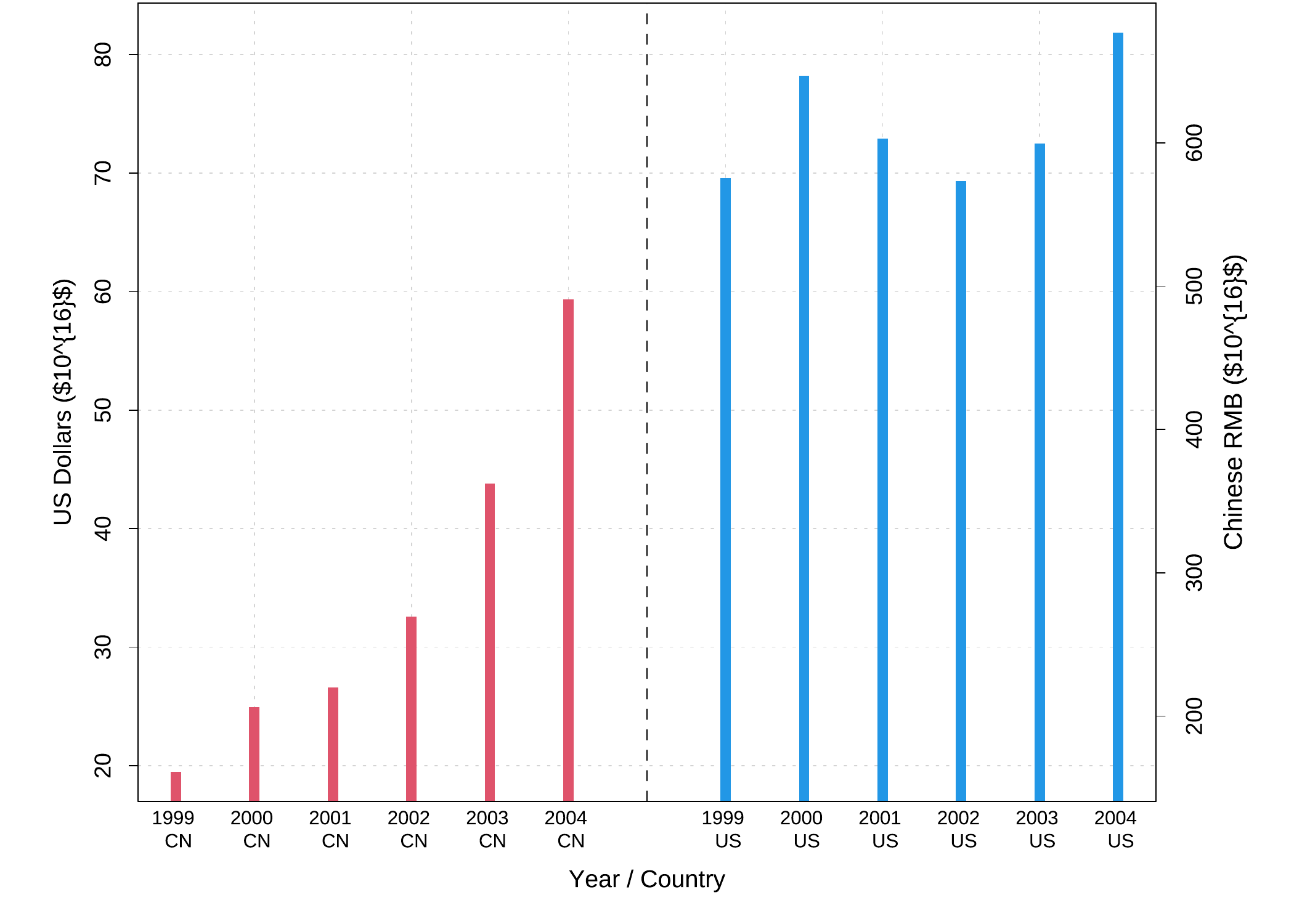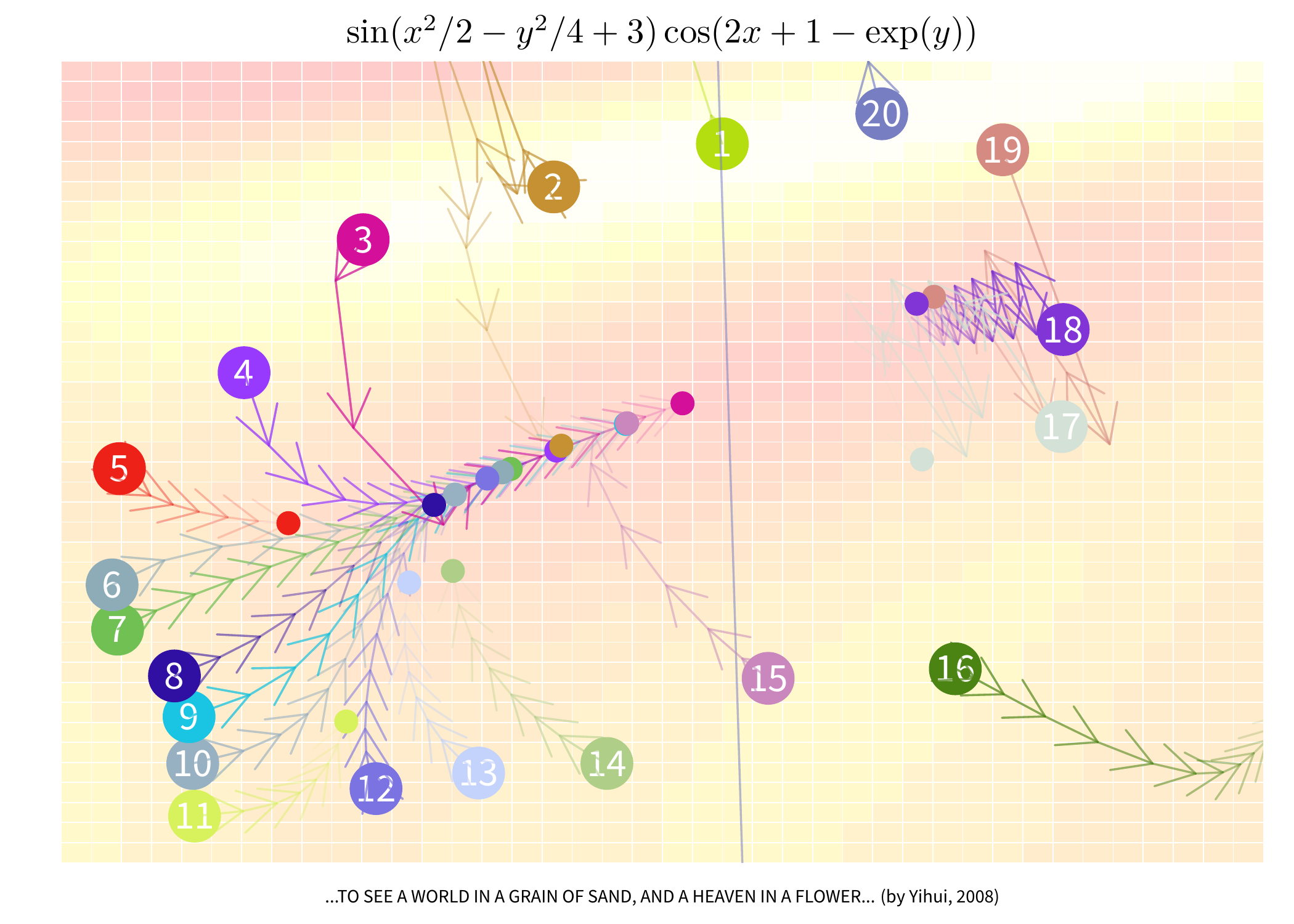# 第 3 章 元素

“现在，”亨利·巴斯克维尔爵士说，“福尔摩斯先生，也许您可以告诉我，这是什么意思，到底是谁对我的事这么感兴趣呢？”

……“可是，亲爱的华生，两者之间的联系非常紧密，短信中的每个单字都是从这个长句中抽出来的。例如：‘你’、‘你的’、‘生’、‘命’、‘理性’、‘价值’、’远离’等，你现在还看不出这些字是从那里面弄来的吗？”

“天啊！太对了！唉呀，您可聪明绝顶！”亨利爵士喊了起来。

— 柯南·道尔《巴斯克维尔的猎犬》

## 3.1 颜色

### 3.1.1 固定颜色选择函数

colors(), colours()

sample(colors(), 20)
##   "plum1"          "lightsalmon3"   "coral"          "lavenderblush"
##   "lavenderblush3" "grey36"         "gray88"         "antiquewhite2"
##   "dimgray"        "darkturquoise"  "gray25"         "grey57"
##  "blue1"          "firebrick1"     "green4"         "cyan3"
##  "gray5"          "salmon1"        "skyblue2"       "seashell3"

pdf("colors-bar.pdf", height = 120)
par(mar = c(0, 10, 3, 0) + 0.1, yaxs = "i")
barplot(rep(1, length(colors())),
col = rev(colors()), names.arg = rev(colors()), horiz = TRUE,
las = 1, xaxt = "n", main = expression("Bars of colors in" ~ italic(colors()))
)
dev.off()
palette()

palette() # 默认的调色板颜色
##  "black"   "#DF536B" "#61D04F" "#2297E6" "#28E2E5" "#CD0BBC" "#F5C710"
##  "gray62"
palette(colors()[1:10]) # 重新设置调色板为 colors() 的前 10 种颜色
palette() # 更改后的调色板颜色
##   "white"         "aliceblue"     "antiquewhite"  "antiquewhite1"
##   "antiquewhite2" "antiquewhite3" "antiquewhite4" "aquamarine"
##   "aquamarine"    "aquamarine2"
palette("default") # 恢复默认调色板

### 3.1.2 颜色生成和转换函数

R 提供了一系列利用颜色生成模型如 RGB 模型（红绿蓝三原色混合）、HSV 色彩模型（色调、饱和度和纯度）、HCL 色彩模型（色调、色度和亮度）和灰色生成模型等。颜色的构造原理比较复杂，超出了本书讨论范围，因此这里仅对相关函数的用法作介绍。

rgb()

hsv()

hcl()

gray(), grey()

gray(seq(0, 1, length = 5))
##  "#000000" "#404040" "#808080" "#BFBFBF" "#FFFFFF"

rgb2hsv()

# 赋值给变量 rgb.mat
(rgb.mat <- matrix(c(255, 0, 0, 0, 255, 0, 0, 0, 255, 10, 100, 200), nrow = 3))
##      [,1] [,2] [,3] [,4]
## [1,]  255    0    0   10
## [2,]    0  255    0  100
## [3,]    0    0  255  200
rgb2hsv(rgb.mat)
##   [,1]      [,2]      [,3]      [,4]
## h    0 0.3333333 0.6666667 0.5877193
## s    1 1.0000000 1.0000000 0.9500000
## v    1 1.0000000 1.0000000 0.7843137
col2rgb()

col2rgb(4) # 调色板中第 4 种颜色默认是蓝色
##       [,1]
## red     34
## green  151
## blue   230
col2rgb("yellow") # 黄色是由红绿混合得到的
##       [,1]
## red    255
## green  255
## blue     0
col2rgb("#FF00FF") # 红蓝混合为紫色
##       [,1]
## red    255
## green    0
## blue   255

### 3.1.3 特定颜色主题调色板

rainbow()

heat.colors()

terrain.colors()

topo.colors()

cm.colors()

library(RColorBrewer)
brewer.pal(9, "Blues")
##  "#F7FBFF" "#DEEBF7" "#C6DBEF" "#9ECAE1" "#6BAED6" "#4292C6" "#2171B5"
##  "#08519C" "#08306B"

layout(matrix(1:3, 3), heights = c(2, 1, 1))
par(mar = c(0, 4, 0, 0))
display.brewer.all(type = "seq") # 连续型：18 种
display.brewer.all(type = "div") # 极端化：9 种
display.brewer.all(type = "qual") # 离散型：8 种### 3.1.4 渐变色的简单原理及应用

xx <- c(1912, 1912:1971, 1971)
yy <- c(min(nhtemp), nhtemp, min(nhtemp))
plot(xx, yy, type = "n", xlab = "Year", ylab = "Temperatures")
for (i in seq(255, 0, -3)) {
yy <- c(45, nhtemp - (nhtemp - min(nhtemp)) * (1 - i / 255), 45) # rgb() 中的绿色成分逐渐变小
polygon(xx, yy, col = rgb(1, i / 255, 0), border = NA)
# 读者可以在这里加上 Sys.sleep(0.05) 以便看清作图过程
}
box() # 补齐边框(x <- rgb(1, seq(0, 1, length = 30), 0))
##   "#FF0000" "#FF0900" "#FF1200" "#FF1A00" "#FF2300" "#FF2C00" "#FF3500"
##   "#FF3E00" "#FF4600" "#FF4F00" "#FF5800" "#FF6100" "#FF6A00" "#FF7200"
##  "#FF7B00" "#FF8400" "#FF8D00" "#FF9500" "#FF9E00" "#FFA700" "#FFB000"
##  "#FFB900" "#FFC100" "#FFCA00" "#FFD300" "#FFDC00" "#FFE500" "#FFED00"
##  "#FFF600" "#FFFF00"
# 读者不妨用 barplot(rep(1, 30), col = x) 看看效果

## 3.2 点

usage(points, "default")
## points(x, ...)

demo("pointTypes", package = "MSG")# 先将鸢尾花的类型转化为整数 1、2、3，便于使用向量
idx <- as.integer(iris[["Species"]])
plot(iris[, 3:4],
pch = c(24, 21, 25)[idx],
col = c("black", "red", "blue")[idx], panel.first = grid()
)
legend("topleft",
legend = levels(iris[["Species"]]),
col = c("black", "red", "blue"), pch = c(24, 21, 25), bty = "n"
)3.5 为本书的一例“彩蛋”：基于随机性的图形可能会具有某种艺术性。这里仅仅展示了四幅可能的输出，其它更多可能的结果参见：https://yihui.org/cn/2010/08/art-of-points-in-r/，或观看演示 demo('pointArts', package = 'MSG')。若读者感兴趣，甚至可以用 R 为自己生成一幅桌面背景图片，制作方法在前面链接中有介绍。

par(mar = c(0.2, 0.2, 0.2, 0.2), mfrow = c(2, 2))
for (n in c(63, 60, 76, 74)) {
set.seed(711)
plot.new()
box()
size <- c(replicate(n, 1 / rbeta(2, 1.5, 4)))
center <- t(replicate(n, runif(2)))[rep(1:n, each = 2), ]
color <- paste("#", apply(
replicate(2 * n, sample(c(0:9, LETTERS[1:6]), 8, TRUE)), 2, paste,
collapse = ""
), sep = "")
points(center, cex = size, pch = rep(20:21, n), col = color)
}## 3.3 曲线、直线、线段、箭头、X-样条

B.1 小节介绍函数 par() 时我们提到了关于线条的一些参数设置，例如 lwdlty 等。类似地，我们可以用函数 lines() 来向图中添加曲线（这里所说的曲线本质上是一些线段的连接，并非光滑的曲线）；下面主要补充说明一下关于线条样式 lty 的设定。

R 中可以实现几乎无数种线条样式，因为它的 lty 参数相当灵活，除了取值 0~6 之外，可以根据一个十六进制的数字串（位数必须是偶数位，且非零）来设定线条的虚实，具体原理是这样：数字串的奇数位上的数字表示画相应长度的实线，然后偶数位上的数字则表示空缺相应的长度，这样就构成了一条虚线。例如，'A5' 表示先画 11 单位长的实线，再接着画 5 单位长的空白，紧接着又画 11 单位长的实线，……，就这样重复下去，完成一条虚线；同理，'711911' 表示：7 单位长实线、1 单位长空白、1 单位长实线、9 单位长空白、1 单位长实线、1 单位长空白。这个十六进制的数字串的最长长度限制为 8 位。

# 不作图，只画出框架，且指定坐标轴范围
plot(1:10, type = "n", xlim = c(0, 10), ylim = c(0, 10))
lines(1:10, abs(rnorm(10))) # 10 个正态随机数绝对值的波动线
abline(a = 0, b = 1, col = "gray") # 不同的直线
abline(v = 2, h = 2, lty = 2)
text(8, 3, "abline(a = 0, b = 1)") # 添加文本
arrows(8, 3.5, 6, 5.7, angle = 40) # 添加箭头
# 参数用了向量：不同灰度的线段
segments(rep(3, 4), 6:9, rep(5, 4), 6:9, col = gray(seq(0.2, 0.8, length = 4)))
text(4, 9.8, "segments")usage(abline)
## abline(a = NULL, b = NULL, h = NULL, v = NULL, reg = NULL, coef = NULL,
##   untf = FALSE, ...)

usage(segments)
## segments(x0, y0, x1 = x0, y1 = y0, col = par("fg"), lty = par("lty"),
##   lwd = par("lwd"), ...)

usage(arrows)
## arrows(x0, y0, x1 = x0, y1 = y0, length = 0.25, angle = 30, code = 2,
##   col = par("fg"), lty = par("lty"), lwd = par("lwd"), ...)

demo("xsplineDemo", package = "MSG")usage(xspline)
## xspline(x, y = NULL, shape = 0, open = TRUE, repEnds = TRUE, draw = TRUE,
##   border = par("fg"), col = NA, ...)

## 3.4 矩形、多边形

R 中绘制多边形也是很方便的，主要使用 polygon() 函数，矩形是多边形的特例，不过 R 也提供了专门的函数 rect() 来绘制它。多边形的主要特征在于增加了一些填充选项，比如颜色填充，或者用阴影线填充。关于颜色、线条样式的设置就不必再重复说明。矩形和多边形的用法如下：

usage(rect)
## rect(xleft, ybottom, xright, ytop, density = NULL, angle = 45, col = NA,
##   border = NULL, lty = par("lty"), lwd = par("lwd"), ...)
usage(polygon)
## polygon(x, y = NULL, density = NULL, angle = 45, border = NULL, col = NA,
##   lty = par("lty"), ..., fillOddEven = FALSE)

x <- rnorm(40) # 产生 40 个正态随机数
plot(x, xlab = "", type = "l") # 画线图
# 请思考为什么坐标值要这样设置：多边形的连线路径
polygon(c(1, 1:40, 40), c(0, x, 0), col = "gray")
xy <- par("usr") # 获取当前图形区域坐标范围，以便下用
# 用白色矩形挡住了 0 以下的部分
rect(xy, xy, xy, 0, col = "white")
lines(x) # 重画一遍 x 的线条
abline(h = 0, col = "lightgray") # 添加水平线xlab = "Year / Country",
ylab = "US Dollars ($10^{16}$)", xaxt = "n", type = "h",
lwd = 10, col = c(rep(2, 6), NA, rep(4, 6)), lend = 1,
panel.first = grid()
)
# 设置 x 轴的刻度标记：\n 的意思是换行符
xlabel <- paste(Export.USCN$Year, "\n", Export.USCN$Country)
xlabel <- ""
abline(v = 7, lty = 2) # 添加一条分隔线
# 使用带有换行符的刻度标记
axis(1, 1:13, labels = xlabel, tick = FALSE, cex.axis = 0.75)
# 换算为人民币再计算另一个坐标轴刻度（汇率 8.27）
ylabel <- pretty(Export.USCN$Export * 8.27) axis(4, at = ylabel / 8.27, labels = ylabel) mtext("Chinese RMB ($10^{16}$)", side = 4, line = 2) box()图 3.11: 双坐标轴图示：中美两国 1999~2004 年出口额，分别以美元和人民币表示。注意图中 x 轴标签文本是如何换行的。 其中，side 参数与 mtext() 函数中的参数意思相类似，表示将坐标轴画在哪条边上，事实上通过前面一些图形元素参数的讲解，读者应该能意识到，R 中上下左右方向的顺序一般都是“下、左、上、右”，分别用 1、2、3、4 表示；at 参数表示在什么位置画坐标轴标记线；labels 参数指定坐标轴刻度标记的字符。 Murrell (2018) 中第 3.4.5 小节举了一个双坐标轴图形的例子，图中用左右两边的纵轴分别表示摄氏和华氏的温度，即：对于图中同一点，既可以对照左边看摄氏度，也可以对照右边看华氏度；这就是双坐标轴的用途。此处我们不妨也举一个类似的例子来说明 axis() 函数的功能。图 3.11 展示的是从 1999~2004 年中美两国的出口贸易总额，其中 axis() 函数主要作用在于两个地方：第一点是横轴的刻度标记，注意这些刻度标记与我们平时看到的标记有所不同，主要是这一系列标记都是两行文本，原因就在于 labels 参数中使用了换行符，对 C 语言比较熟悉的读者对此不会感到陌生，这里第一行是年份，第二行说明了国家：CN 表示中国，US 表示美国；第二点是图的右边多了一根坐标轴，左边的纵轴表示出口额按美元计价（单位：$$10^{16}$$ 美元，出口额数值太大，因此采用较大的单位），右边表示同样的出口额用人民币计价（例如右边轴上 400 人民币对应左边 $$400/8.27\approx48.4$$ 美元），同一个数值在图中既可以从左边观察美元金额，又可以从右边观察人民币金额。本例中使用的数据来源为：汇率数据来自联合国统计署网站 https://unstats.un.org，从 1999~2004 年人民币和美元的平均汇率稳定在 8.27，出口额年度数据来自 WTO 网站：https://data.wto.org。整理后的数据如下，程序代码可以参见图 3.11 data(Export.USCN, package = "MSG") Export.USCN ## Export Year Country ## 1 19.4931 1999 CN ## 2 24.9203 2000 CN ## 3 26.6098 2001 CN ## 4 32.5596 2002 CN ## 5 43.8228 2003 CN ## 6 59.3326 2004 CN ## 7 NA NA <NA> ## 8 69.5797 1999 US ## 9 78.1918 2000 US ## 10 72.9100 2001 US ## 11 69.3103 2002 US ## 12 72.4771 2003 US ## 13 81.8520 2004 US ## 3.9 图形元素应用示例 在介绍了图形的基本构成元素的作法之后，我们应该可以理解 R 作图的自由——几乎所有图形的细节都可以被我们控制。下面我们举几个例子来说明如何利用图形元素来构造完整的图形。 ### 3.9.1 瀑布图 瀑布图（Waterfall Chart）的最初发明者我们无法考证，但可以在麦肯锡的报告中看到它的身影。如果在 Google 中搜索 Excel 瀑布图，我们可以看见一些令人无奈的结果，例如 步步致赢瀑布图(步行图)制作系统 1.1 […] 下载 […] 共享版最多支持 6 个数，正式版无限制。 或是 瀑布图的完美解决方案 [Excel 图表] […] 简单说明一下：[…] （分为 6 步） 作者曾在某咨询公司听过一场关于 Excel 作图的培训，其中也大谈瀑布图的技巧，大致思路是用一些本来不直观的方法来完成瀑布图的制作，例如用白色填充矩形条，使之“隐藏”起来，而上面的矩形条才能得到“悬空”的效果。 瀑布图究竟是什么呢？只不过是一系列矩形而已（3.4 小节）。它的外观类似于条形图，区别在于除了第一个矩形条之外，后面的矩形条都不再以零点为基准线来画，而是以前一个矩形条的高度为起点画矩形到该矩形条位置上的取值，这样可以反映一个变量取值的上下变化情况（主要是体现相邻矩形条的差值）。下面我们结合一个数据实例来说明瀑布图的简单思想和简单做法。 我们选取了 2010 年 8 月第一周“统计之都”网站的“绝对唯一访问次数”（Absolute Unique Visitors）来画瀑布图，数据如下 # 七天之内的唯一访问次数数据（周一为 2010 年 8 月 2 日） auv <- c(939, 1005, 973, 910, 875, 658, 688) # 相邻两天作差 diff(auv) ##  66 -32 -63 -35 -217 30 瀑布图中第一个矩形条高度为 939，第二个矩形则从 939 继续向上画 $$1005-939=66$$ 单位，第三个矩形则从 1005 开始向下画 32 单位，依此类推。对于访问量下滑的那些日期，可以用特殊颜色标示（比如红色警告），以区分访问量增长的日期。图 3.12 给出了 R 代码和瀑布图结果，其中核心代码只有最后一句，前面的代码仅以布局和美观为目的。从代码可以看到，我们用低层作图函数 rect() 来画瀑布图是非常直观的：前四个参数给定了矩形的位置坐标，而这些位置经过简单的逻辑思考很容易得到。所有任务只是画矩形而已，在缺少对图形元素充分支持的软件包中，我们付出了不必要的代价。 par(mar = c(4, 4, .5, .1)) plot(auv, xlab = "八月第一周", ylab = "绝对唯一访问次数", type = "n", xlim = c(0.5, 7.5), ylim = c(0, max(auv)), xaxt = "n", panel.first = grid() ) axis(1, 1:7, sprintf("周%s", c( "一", "二", "三", "四", "五", "六", "日" ))) rect(1:7 - 0.3, c(0, auv[1:6]), 1:7 + 0.3, auv, col = c(NA, ifelse(diff(auv) < 0, "red", NA)) )图 3.12: “统计之都”网站八月第一周访问数据瀑布图：周一访问量为 939， 周二上升，周三开始下降（红色矩形表示访问量下降），直到周末降到最低。这个规律在其它星期都存在，也许它反映了大众普遍的劳累和忙碌规律？ ### 3.9.2 梯度下降算法 箭头在图形中的作用通常是指示，例如图 3.6 中的箭头用来将文本标注指向一条直线，这样使得文本的位置可以灵活安排。下面我们再给一个看起来更复杂的例子，来说明箭头的一个自然而然应用。很多统计计算过程都涉及到迭代，因为这些计算问题没有显式解（即：没有明确的数学表达式），很难一步得到答案，但可以通过迭代来一步步逼近真实解，梯度下降算法就是诸多具有迭代特征方法中的一种。梯度下降算法的目标在于寻找一个函数 $$F(\mathsf{x})$$ 的极值，不失一般性，我们假设寻找的是极小值。这里我们简单描述一些梯度下降算法的原理： 假设函数 $$F(\mathsf{x})$$ 在点 $$\mathsf{a}$$ 的一个小邻域内可导（这里 $$\mathsf{x}$$ 可以是多维向量），那么从点 $$\mathsf{a}$$ 出发，$$F(\mathsf{x})$$ 沿负梯度方向 $$-\nabla F(\mathsf{a})$$ 函数值下降最快。可以证明对足够小的 $$\gamma>0$$，若 $\mathsf{b} = \mathsf{a}-\gamma\nabla F(\mathsf{a})$ 那么 $$F(\mathsf{a})\geq F(\mathsf{b})$$。这样，我们随机猜测一个迭代的起点 $$\mathsf{x}_{0}$$，然后按以下方式迭代计算下一步的解 $$\mathsf{x}_{n}$$ $\mathsf{x}_{n+1} = \mathsf{x}_{n}-\gamma\nabla F(\mathsf{x}_{n}),\ n\ge0$ 即可满足： $F(\mathsf{x}_{0})\ge F(\mathsf{x}_{1})\ge F(\mathsf{x}_{2})\ge\cdots$ 这样序列 $$\left\{ \mathsf{x}_{n}\right\}$$ 将收敛到局部最小值。 上述算法中，核心计算就是目标函数 F 的导数（多维情况下通常称为梯度），剩下的就是简单的四则运算。求导可以用 R 自身的函数 deriv()，所以这个计算实现起来并不困难，而我们想要通过一个例子来说明几个在统计计算中容易被广大统计应用者忽略的问题： 1. 迭代的起点 2. 步长（本算法中可以看作是 $$\gamma$$ 3. 迭代次数 4. 误差 我们在实际应用中，往往过于依赖和相信计算机程序，认为所有默认设置都是最优或最可靠的，实际并非如此，否则所有的函数都没有必要设置参数了。以上四个问题是大多数迭代性质的算法都会涉及到的：迭代起点通常需要和真实的解相隔较近，但这个要求不具备可操作性，因为我们并不知道真实解在哪里；步长通常尽量小，保证迭代能稳定收敛，否则迭代过程中跳跃很大不容易找到解，但小步长的缺点也很明显，它使得迭代进行得很慢；迭代次数通常需要尽量大；误差显然应该越小越好，例如在求根的问题中，我们希望目标函数值越接近于 0 越好。图 3.13 选取了一个二元函数 $f(x,y)=\sin(x^{2}/2-y^{2}/4+3)\cos(2x+1-\exp(y)),$ 目标是在 $$\left\{ (x,y)|x\in[-1,1],\ y\in[0.5,2.2]\right\}$$ 范围内求得一对 $$(x,y)$$ 使得 $$f(\cdot,\cdot)$$ 最小，整幅图的背景是一幅颜色图（4.14 小节将会介绍），图中横轴表示 x，纵轴表示 y，每一对 $$(x,y)$$ 对应的地方有一个颜色方块，它的颜色深浅代表了函数 $$f(x,y)$$ 的取值大小，越深表示值越小。理想情况是，我们随意选取一个迭代起点，梯度下降算法能把我们带到图中的最深处去，这个过程在图中用一系列首尾相接的箭头表示，但图 3.13 显示的情况并不完全与我们的预期相符：有些起始点能把我们带到低处，有些则不能；有些步长下收敛较稳定，有些步长则让迭代路径蜿蜒崎岖；有的箭头还没到最低点就停滞不前了，有些则一直能往最低的地方走。本例的目标函数也可以用 R 自身的 optim() 函数来求极小值，它的收敛代码为 0，表示这个计算结果在一定条件下可以收敛（但不代表找到了全局最小值，甚至不代表找到了局部最小值）： f2 <- function(x) sin(1 / 2 * x^2 - 1 / 4 * x^2 + 3) * cos(2 * x + 1 - exp(x)) # par 为局部解；value 为目标函数值； convergence 为收敛指示代码 optim(c(-.9, .9), f2, method = "L-BFGS-B", lower = c(-1, .5), upper = c(1, 2.2)) ##$par
##  0.3227365 1.6024052
##
## $value ##  -0.6574 ## ##$counts
##       13       13
##
## $convergence ##  0 ## ##$message
##  "CONVERGENCE: REL_REDUCTION_OF_F <= FACTR*EPSMCH"

3.13 同时展示了梯度下降算法的原理以及在应用中可能出现的问题（演示 demo('gradArrows2', package = 'MSG') 可以获得更好的图形质量以及箭头动画效果），它充分显示了 R 语言的统计图形能力可以紧密结合它的统计计算优势，创造出有意义的统计图形。我们使用的只是简单的图形元素（箭头）和基本的计算（求导），但结果的深刻远大于过程的简单。从某种程度上来说，在 R 语言中作图缺的不是工具（工具俯拾皆是），而是创意、思考以及对数据的理解。

demo("gradArrows1", package = "MSG")demo("signSTAT", package = "MSG")## 3.11 思考与练习

1. 彩色图形虽然在外观上很吸引人，但是它的一个局限是可能受客观条件限制而不能被打印出来（例如黑白打印机），我们在选取颜色的时候该如何平衡外观和打印效果？另外，男性和女性的色盲 / 色弱比例各是多少？（可以查阅维基百科：https://en.wikipedia.org/wiki/Color_blindness

2. 3.4 在点的样式选取上是否有明显的缺陷？或者说散点图中的点应该如何选取形状以使得各组点之间的外观差异尽量大？第 7.1.2 小节会对这个问题有详细评论。

3. 当箭头角度参数 angle$$90\,^{\circ}$$code 参数为 3 时，箭头的形状是怎样的？这种形状是否和数学上的“区间”有类似之处？请考虑如何利用这种“双箭头”表达统计学中的置信区间。

4. 利用三角形的边长关系画一个指北箭头如下5. 一些数学上函数可以产生有趣的“心形曲线”，例如在极坐标系下 $$r(\theta)=1-\sin(\theta)$$ 生成的就是心形的曲线，笛卡尔坐标下方程 $$(x^{2}+y^{2}-1)^{3}-x^{2}y^{3}=0$$ 对应的解也可以形成心形曲线。这里我们考虑一种简单的心形曲线如下图所示：这是个左右对称的形状，因此只需要知道左右任一侧的画法就知道整体画法了。实际上，右侧由上下两部分构成，上面是直径为 1 的半圆，下面是翻转了 $$90\,^{\circ}$$ 的曲线 $$y=\sin(x)，x\in[0,\pi/2]$$。请思考如何用多边形构造这个形状。MSG 包中的函数 heart_curve() 可供参考。

6. 温度计形状用来表达比例数据通常很形象，我们可以设定温度计的范围在 $$[0,\,1]$$ 之间，用汞柱的高度来表达比例。用 R 构造一支“温度计”很简单，如下图：这些温度计实际上由一个灰圆点、一个红圆点、一条灰线段和一条红线段叠加构成，红线段的长度表示比例大小，其它图形元素都只是背景。请尝试用四个图形元素构造温度计，注意这些图形元素的叠加顺序（例如红线必须在灰线之后画，否则会被覆盖）。4.26 小节的符号图中，“符号”的选项之一就是用温度计，但不如这里的温度计形象。对于追求细节完美的用户来说，这里线段末端的样式也值得注意，因为我们实际生活中看到的汞柱末端是弧形的，所以这里要是画成矩形就不合适了；线段末端的三种样式在 B.1 小节中有介绍。### 参考文献

Murrell, Paul. 2018. R Graphics. 3rd ed. Boca Raton, Florida: Chapman; Hall/CRC. https://www.stat.auckland.ac.nz/~paul/RG3e/.
Neuwirth, Erich. 2014. RColorBrewer: ColorBrewer Palettes. https://CRAN.R-project.org/package=RColorBrewer.
———. 2013. animation: An R Package for Creating Animations and Demonstrating Statistical Methods.” Journal of Statistical Software 53 (1): 1–27. https://www.jstatsoft.org/v53/i01/.